Electric Charge Key Question How do electric charges

• Slides: 12Electric Charge Key Question: How do electric charges interact?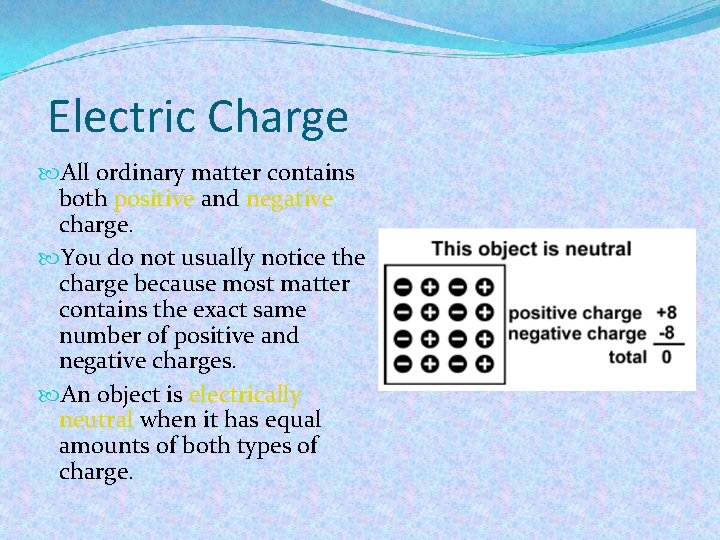Electric Charge All ordinary matter contains both positive and negative charge. You do not usually notice the charge because most matter contains the exact same number of positive and negative charges. An object is electrically neutral when it has equal amounts of both types of charge.Electric Charge Objects can lose or gain electric charges. The net charge is also sometimes called excess charge because a charged object has an excess of either positive or negative charges. A tiny imbalance in either positive or negative charge on an object is the cause of static electricity.Electric Charge Electric charge is a property of tiny particles in atoms. The unit of electric charge is the coulomb (C). A quantity of charge should always be identified with a positive or a negative sign.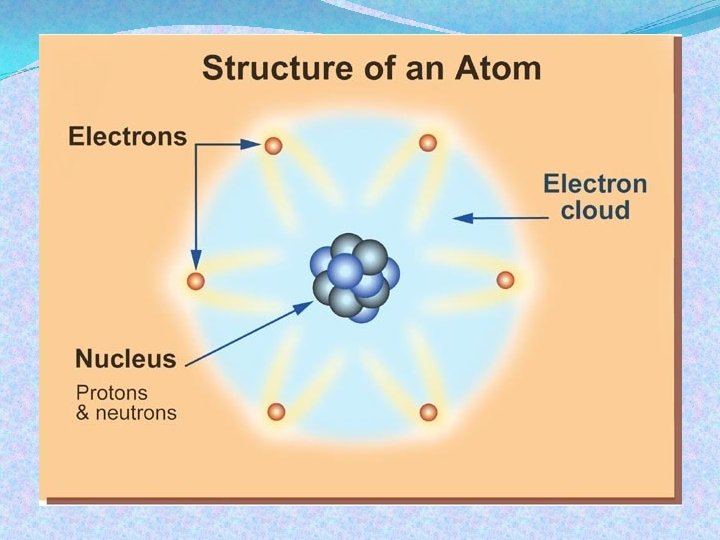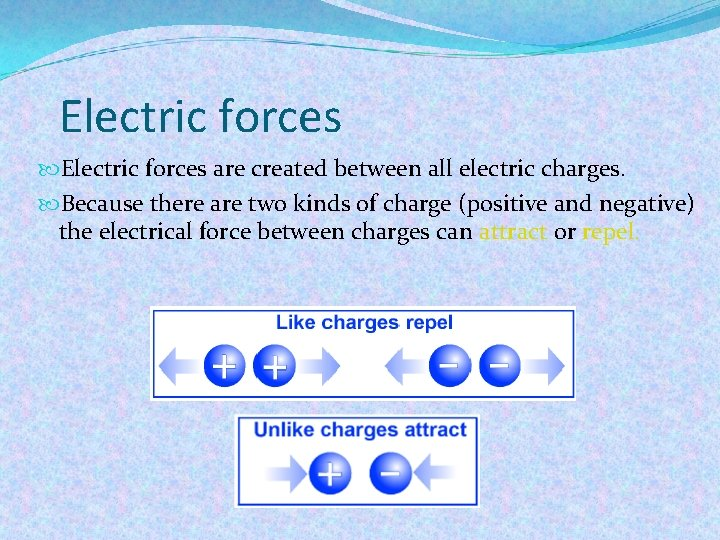Electric forces are created between all electric charges. Because there are two kinds of charge (positive and negative) the electrical force between charges can attract or repel.Coulomb's Law Coulomb’s law relates the force between two single charges separated by a distance. Constant 9 x 109 N. m 2/C 2 Force (N) F = K q 1 q 2 Charges (C) r 2 Distance (m)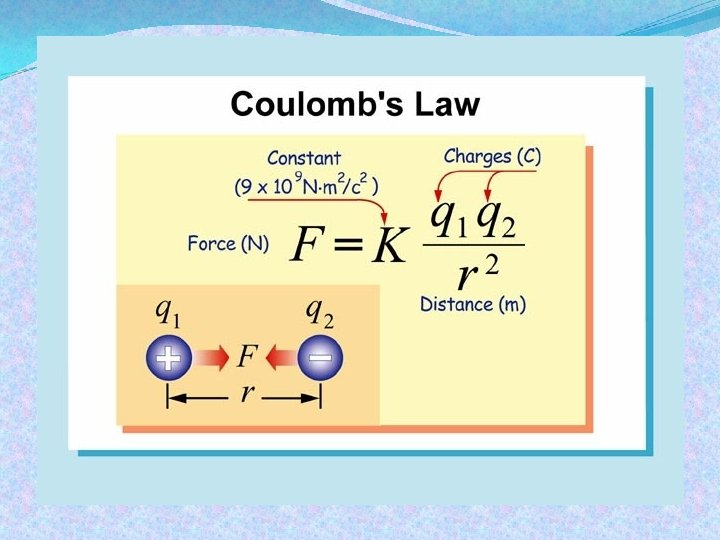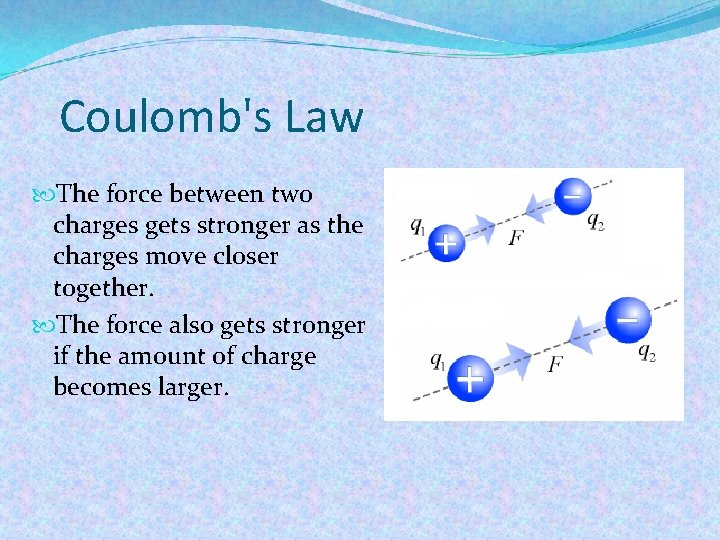Coulomb's Law The force between two charges gets stronger as the charges move closer together. The force also gets stronger if the amount of charge becomes larger.Coulomb's Law The force between two charges is directed along the line connecting their centers. Electric forces always occur in pairs according to Newton’s third law, like all forces.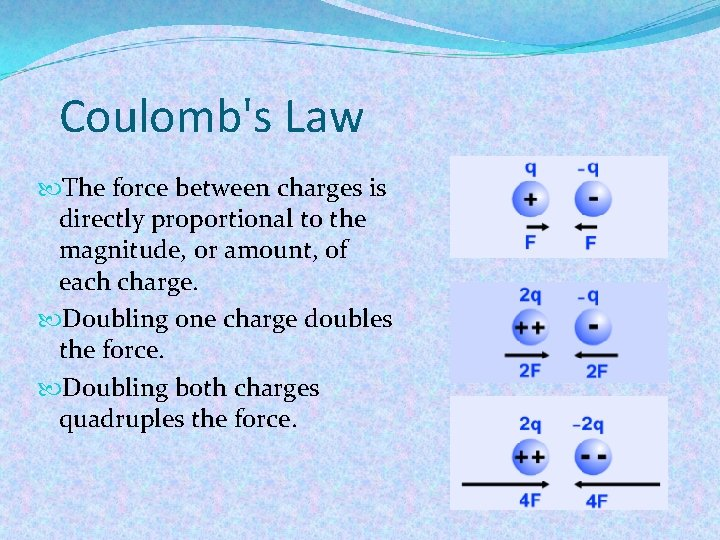Coulomb's Law The force between charges is directly proportional to the magnitude, or amount, of each charge. Doubling one charge doubles the force. Doubling both charges quadruples the force.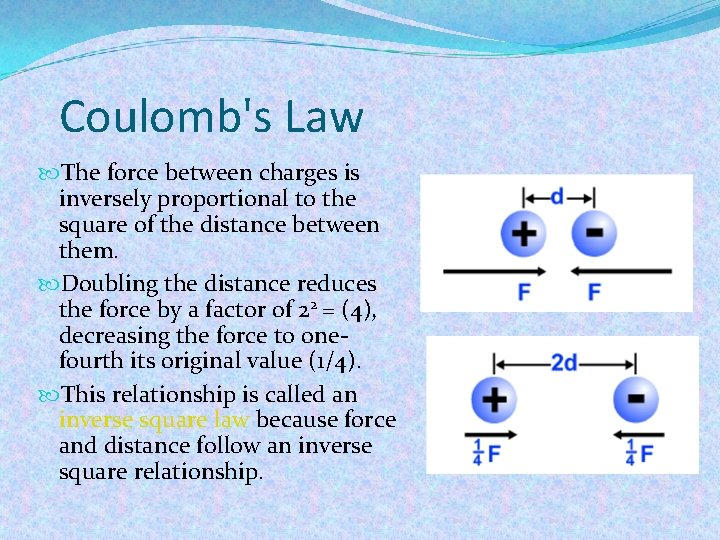Coulomb's Law The force between charges is inversely proportional to the square of the distance between them. Doubling the distance reduces the force by a factor of 22 = (4), decreasing the force to onefourth its original value (1/4). This relationship is called an inverse square law because force and distance follow an inverse square relationship.